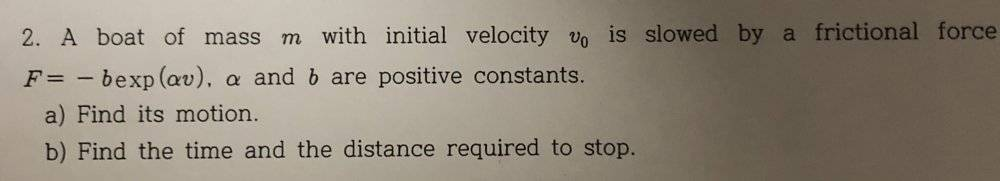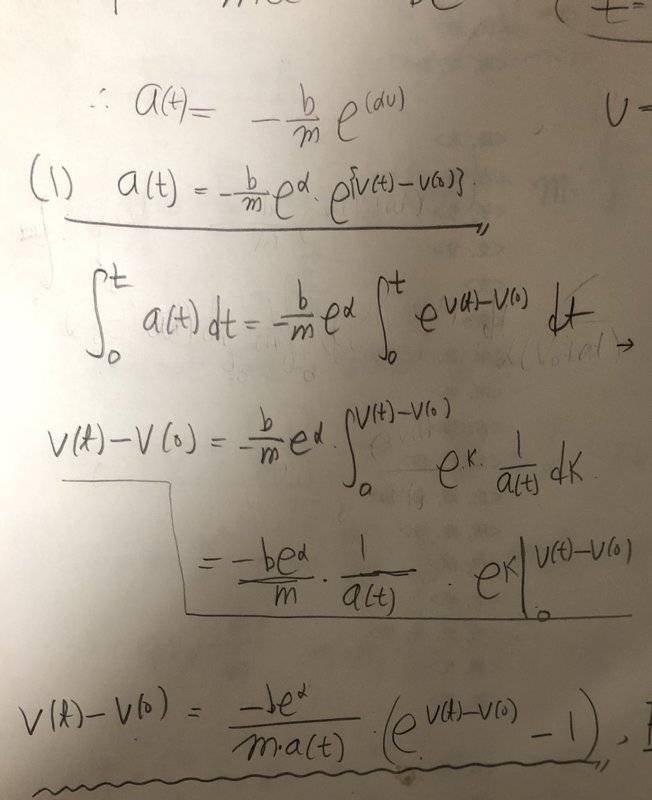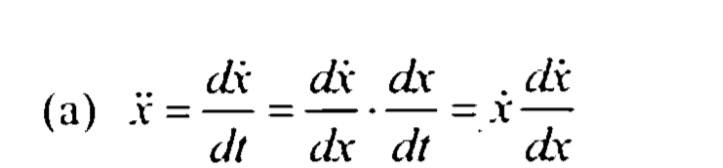# Rectilinear motion, not uniform accleration

• Terrycho

#### Terrycho

Homework Statement
A boat of mass m with initial velocity v_o is slowed by a frictional force
F=-bexp(αv), α and b are positive constants .
Relevant Equations
F=-bexp(αv)The photo above is the problem I'm struggling now and the photo below is what I have done so far.By differentiating and integrating the given equation, which is F=-b exp(αv), I tried to express v(t) or a(t) as a function related to t, which is time.

But the more I did, the more complicated it got...

Force is related to the velocity so when you differentiate it, the acceleration function about t comes out so you cannot just express its motion.

How was I supposed to approach this problem to?

Look forward to hearing from you guys.
Thanks a lot!

If this posts makes any inconvenience or you offended, I will delete it right away.

Hello terycho, ##\qquad####\qquad## !

Do us a favor and read the guidelines on posting photos. If you promise to type instead of snap I'll try to help:

Don't understand your first step: you multiply with ##e^{-v(0)}\ ## on one side only: no can do.

If you rewrite ##\ a(t) = - {b\over m} e^{\alpha v} \ ## as ##\ {dv\over dt} = - {b\over m} e^{\alpha v} \ ## does it look a bit more familiar ?
Do you know how to solve this kind of differential equation ?
(hit: think chain rule for differentiation)

Hello terycho, ##\qquad####\qquad## !

Do us a favor and read the guidelines on posting photos. If you promise to type instead of snap I'll try to help:

Don't understand your first step: you multiply with ##e^{-v(0)}\ ## on one side only: no can do.

If you rewrite ##\ a(t) = - {b\over m} e^{\alpha v} \ ## as ##\ {dv\over dt} = - {b\over m} e^{\alpha v} \ ## does it look a bit more familiar ?
Do you know how to solve this kind of differential equation ?
(hit: think chain rule for differentiation)
I modified the post and read the guide! Sorry for breaking the rules.. My bad

And I know how to solve this kind of equations. We need to use this formula(attached the photo below). I read the formula you rewrote, but isn’t v also related to time? If I move dv to the right and do integration , it gets much more complexed since v is also the function related to time

#### Attachments

Last edited:
I modified the post and read the guide! Sorry for breaking the rules.. My bad

And I know how to solve this kind of equations. We need to use this formula(attached the photo below). I read the formula you rewrote, but isn’t v also related to time? If I move dv to the right and do integration , it gets much more complexed since v is also the function related to time
You don't "move" anything, especially you can't move ## dv ##.

Use the form of the equation suggested by @BvU . ##\displaystyle \quad \ {dv\over dt} = - {b\over m} e^{\alpha v} ##

Divide both sides by ## e^{\alpha v} ## and integrate with respect to ##t## .

Note that ##\displaystyle \int f(v(t)) \frac{dv}{dt} dt = \int f(v) dv \ \ \ ## (Integration by substitution)

•Terrycho
You don't "move" anything, especially you can't move ## dv ##.

Use the form of the equation suggested by @BvU . ##\displaystyle \quad \ {dv\over dt} = - {b\over m} e^{\alpha v} ##

Divide both sides by ## e^{\alpha v} ## and integrate with respect to ##t## .

Note that ##\displaystyle \int f(v(t)) \frac{dv}{dt} dt = \int f(v) dv \ \ \ ## (Integration by substitution)
I didn’t think of dividing both sides by e^(av). I solved this problem! Thanks a lot!

I modified the post and read the guide! Sorry for breaking the rules.. My bad

And I know how to solve this kind of equations. We need to use this formula(attached the photo below). I read the formula you rewrote, but isn’t v also related to time? If I move dv to the right and do integration , it gets much more complexed since v is also the function related to time
I solved it! Thanks!

...
And I know how to solve this kind of equations. We need to use this formula(attached the photo below). I read the formula you rewrote, but isn’t v also related to time?This formula is useful in the case where acceleration is given as some function of position or velocity, and in some cases both.

In your case it can be used to to find velocity as a function of position.

The equation ##\
\displaystyle \quad \ {dv\over dt} = - {b\over m} e^{\alpha v} \
## can be written as:

## \displaystyle \quad \ v{dv\over dx} = - {b\over m} e^{\alpha v} ##​

Dividing by ##\ e^{\alpha v} \ ## and integrating with respect to position, ##x## give the following,

## \displaystyle \int v \ e^{-\alpha v} \left({dv\over dx}\right) dx = - \int {b\over m} dx ##